# R Weekly Bulletin Vol – VIII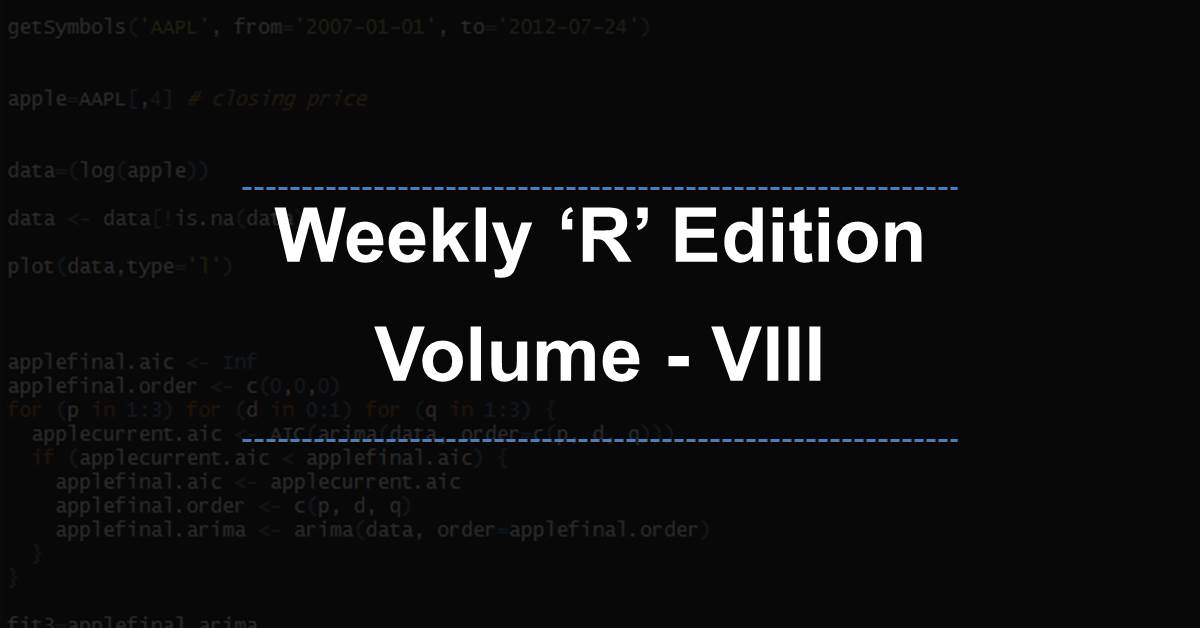This week’s R bulletin will cover topics on plotting charts like saving the plot, adding a grid, and plotting multiple data sets in a single plot. Hope you like this R weekly bulletin. Enjoy reading!

### Shortcut Keys

1. Run current document - Ctrl+Alt+R
2. Run from document beginning to current line - Ctrl+Alt+B
3. Run from current line to document end - Ctrl+Alt+E

### Problem Solving Ideas

#### Saving a plot to a file

R allows you to save a plot in different file formats, such as PNG, JPEG, or PDF. The example below outlines the process for saving plots in R.

Example: We would like to generate a 2-year closing price series plot for BPCL, and then save the plot in a PNG file. We first call the png function and provide the desired filename, width, and height of the plot. We then plot the price series along with the desired arguments to the plot function. Finally, we close the graphics file using the dev.off function. The file is saved in the current working directory unless you specify some other desired path.

library(quantmod)
bpcl = getSymbols("BPCL.NS", src = "yahoo", from = "2014-01-01", to = "2016-01-01",
auto.assign = FALSE)

bpcl_cl = Cl(bpcl)

plot.ts(bpcl_cl, main = "BPCL Price Series", xlab = "Days", ylab = "Close Price",
type = "l", col = "red")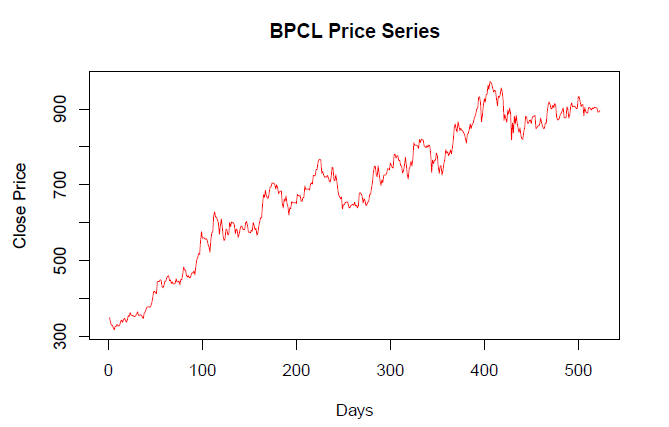# Call the png function, Plot the vectors, and finally close the graphics file
png("Saving plot.png", width = 680, height = 480)
plot.ts(bpcl_cl, main = "BPCL Price Series", xlab = "Days", ylab = "Close Price",
type = "l", col = "red")
dev.off()

pdf 2

# Saving the plot in pdf

pdf("Saving plot.pdf")
plot.ts(bpcl_cl, main = "BPCL Price Series", xlab = "Days", ylab = "Close Price",
type = "l", col = "red")
dev.off()

pdf 2

#### Adding a grid to the plot

A grid can be added to a plot using the grid function. To plot a grid, we first call the plot function with type=“n” to initialize the graphics frame without displaying the data. In the next step, we call the grid function to draw the grid. Finally, we call the lines function to draw the graphics overlaid on the grid. If we want to have point instead of a line, we can use the points function.

Example:

library(quantmod)
techm = getSymbols("TECHM.NS", src = "yahoo", from = "2015-01-01", to = "2016-01-01",
auto.assign = FALSE)
techm_cl = coredata(Cl(techm))
days = index(Cl(techm))
# Adding grid to a line chart

plot(days, techm_cl, main = "TECHM Price Series", xlab = "Days", ylab = "Close Price", type = "n")
grid(col = "red", lwd = 1.5)
lines(days, techm_cl)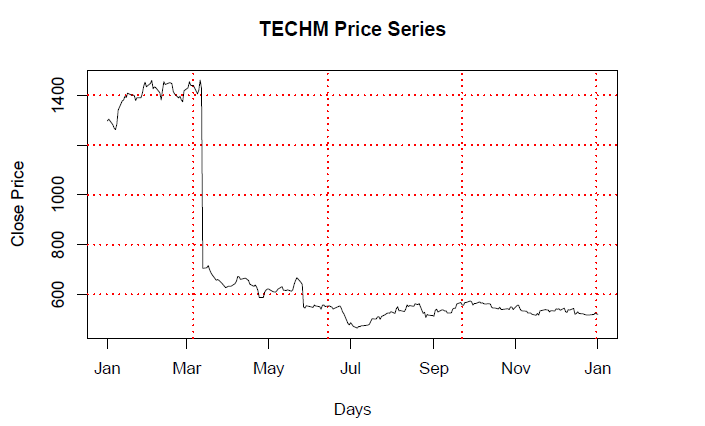# Adding grid to a point chart

plot(days, techm_cl, main = "TECHM Price Series", xlab = "Days", ylab = "Close Price", type = "n")
grid(col = "blue", lwd = 1.5)
points(days, techm_cl)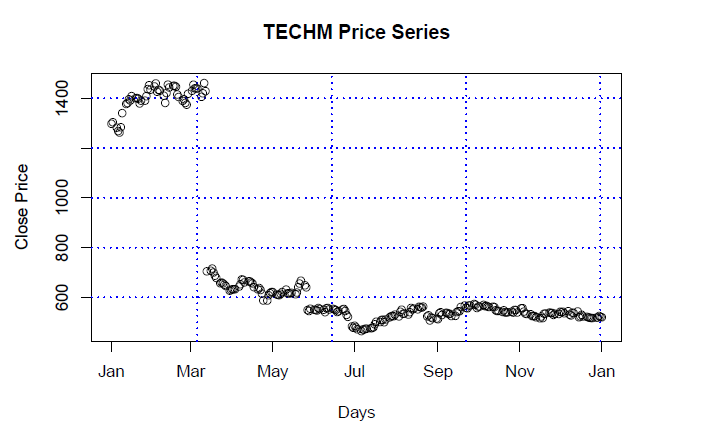#### Plotting Multiple Datasets

To plot multiple datasets we use a high-level function like plot, followed by a low-level function like the “lines” function. Since multiple datasets can have different X-axis and Y-axis ranges, it is important to set the range for the two axes in such a way that the plot incorporates all the data points from the multiple datasets.

In the example below, we are plotting the 1-year daily closing price series for two stocks, namely PNB and CANBK. We use the range function to determine the xlim and ylim parameters. Calling the range function on the close price series of the two datasets ensures that we plot all the data points. Thereafter we call the high-level “plot” function on the closing price series of PNB stock and defined the other necessary parameters. We then use the low-level “lines” function to add the closing price series for CANBK stock.

Example:

library(quantmod)
pnb = getSymbols("PNB.NS", src = "yahoo", from = "2015-01-01", to = "2015-12-31",
auto.assign = FALSE)

canbk = getSymbols("CANBK.NS", src = "yahoo", from = "2015-01-01", to = "2015-12-31",
auto.assign = FALSE)

pnb_close = coredata(Cl(pnb))
canbk_close = coredata(Cl(canbk))
date = index(pnb)

main = "PNB-CANBK Daily Close Price Chart for 2015"
xlim = range(as.Date(date))
ylim = range(c(pnb_close, canbk_close))

plot(date, pnb_close, type = "l", lty = 1, pch = 19, col = "red", xlab = "Months",
ylab = "Price", main = main, xlim = xlim, ylim = ylim)# Add a line
lines(date, canbk_close, type = "l", lty = 1, pch = 18, col = "blue")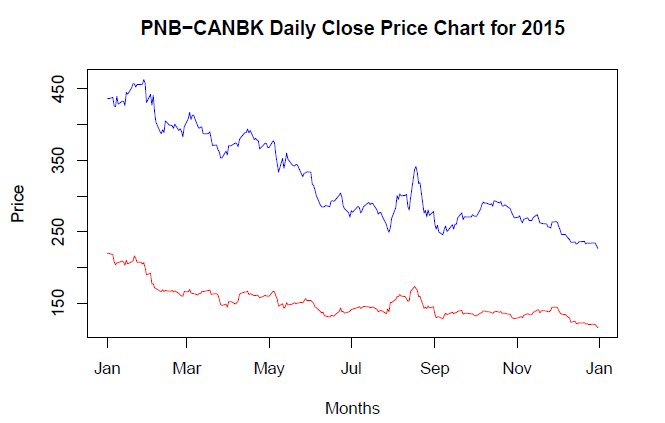### Functions Demystified

The download.file function helps download a file from a website. This could be a webpage, a csv file, an R file, etc. The syntax for the function is given as:

where, url - The Uniform Resource Locator (URL) of the file to be downloaded destfile - the location to save the downloaded file, i.e. path with a file name

Example: In this example, the function will download the file from the path given in the “url” argument, and saved it in the D drive within the “Skills” folder with the name “betawacc.xls”.

url = "http://www.exinfm.com/excel%20files/betawacc.xls"
destfile = "D:/Skills/wacc.xls"
download.file(url, destfile)

#### file.copy and file.rename

The file.copy function copies a file from one folder to another folder, while the file.rename function renames an existing file in a given folder.

Example: The path of the file to be copied is mentioned as the first argument, and the location to copy is mentioned as the second argument in the function.

file.copy("C:/Users/MyFolder/TATACHEM.csv", "D:/Documents/TATACHEM.csv")

Example: The path of the file to be renamed is mentioned as the first argument, and the location of the file after renaming is mentioned as the second argument in the function. The syntax is given as:

file.rename(from=(path of the file), to=(path of the file))

#### file.exists and file.remove

The file.exists function is used to check whether a particular file is present in the set working directory or other folder specify as the argument to the function. The file.remove function is used to delete a particular file if it is present in the set working directory.

Example: This will check whether the file “TATACHEM.csv” exists in the “MyFolder” folder.

file.exists("C:/Users/MyFolder/TATACHEM.csv")
 TRUE

This will delete the “TATACHEM.csv” file from the “MyFolder” folder.

file.remove("C:/Users/MyFolder/TATACHEM.csv")

### Next Step

We hope you liked this bulletin. In the next weekly bulletin, we will list more interesting ways and methods plus R functions for our readers.

Update

We have noticed that some users are facing challenges while downloading the market data from Yahoo and Google Finance platforms. In case you are looking for an alternative source for market data, you can use Quandl for the same.